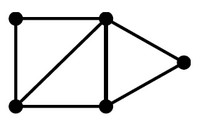# Tree - Visualization

A tree can be rendered with all graph visualization libraries.

List
• Rectangular Treemap
• Sunburst Tree. A sunburst is similar to the treemap, except it uses a radial layout.
• Sunray Tree
• Iceray Tree
• Hyperbolic Tree
• Diagonal Tree

Large data set:

• fractal maps,
Pyramid

A pyramid or triangle diagram where:

• the height of a level represents that level's status
• and width of a level represents the quantity of items at that level relative to the whole.

For example:

• An organizational chart is the diagram of a hierarchy within an organization
• Maslow's hierarchy of human needs
Library
Documentation / Reference

Recommended Pages(Graph|Network) - Visualization

Graph visualization is based on the mathematical theory of networks, graph theory. Chord graph. Nodes are place on a circle. Death Star dependency graph Radial, diagonal and Dendro Network...Web UI - Tree View

A tree visualization React: CoffeeScript: Bootstrap: (deprecated)...